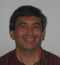Miroslav Chlebik
Course convenor, MSc Mathematics
E:
T: +44 (0)1273 877446

Research

• Nonlinear partial differential equations
• Calculus of variations
• Geometric measure theory
• Classical real analysis
• Combinatorial optimization
• Computational complexity